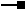# IAffineTransformation2D3 Interface

## Members

Name DescriptionDefineConformalFromControlPoints Defines the best conformal affine transformation between two sets of points. Can be used to register paper maps on a digitizer.DefineConformalFromControlPointsEx Defines the best conformal affine transformation between two sets of points. Can be used to register paper maps on a digitizer.DefineFromControlPoints Defines the best affine transformation between two sets of points. Can be used to register paper maps on a digitizer.DefineFromControlPointsEx Defines the best affine transformation between two sets of points. Can be used to register paper maps on a digitizer.DefineFromEnvelopes Defines a transformation that maps a point relative to one envelope to a similar position relative to another envelope.DefineFromEnvelopesEx Defines a transformation that maps a point relative to one envelope to a similar position relative to another envelope.DefineReflection Defines a transformation that can perform a reflection about the line l.GetControlPointError Returns the errors involved in moving control point i from the 'from' to 'to' system. These error terms are valid after using DefineFromControlPoints/Ex to define the transformation.GetRMSError RMS (Root Mean Square) error expressed relative to the 'from' and 'to' points defining the transformation. These error terms are valid after using DefineFromControlPoints/Ex to define the transformation.IsReflective Indicates if the transformation contains a reflection (determinant is negative).Move Incorporates a translation factor into the transformation.MoveOrigin The origin of accumulated transformations used when projecting an affine transformation to a different spatial reference system.MoveOrigin The origin of accumulated transformations used when projecting an affine transformation to a different spatial reference system.MoveVector Performs an X and Y translation defined by a 2D vector.PostMultiply Post-multiplies the transformation by another transformation.PreMultiply Pre-multiplies the transformation by another transformation.Project Moves this transformation into another spatial reference. If the transformations contains only translations, then use the MoveOrigin property to define an equivalent translation in the new spatial reference.QueryLinearCoefficients Returns the linear coefficients which define the two dimensional affine transformation.Reset Resets the tranformation.Rotate Incorporates a rotation (in radians) into the transformation.Rotation The rotation angle. Will not be able if different x/y scale factors have been incorporated into the transformation.Scale Incorporates scale factors into the transformation.SetLinearCoefficients Sets the linear coefficients which define the two dimensional affine transformation.SpatialReference The spatial reference in which this transformation is meaningful.TransformMeasuresFF Transforms floating point measures to floating point measures (or do the inverse).TransformMeasuresFI Transforms floating point measures to integer measures (or do the inverse).TransformMeasuresIF Transforms integer measures to floating point measures (or do the inverse).TransformMeasuresII Transforms integer measures to integer measures (or do the inverse).TransformPointsFF Transforms floating point points to floating point points (or do the inverse).TransformPointsFI Transforms floating point points to integer points (or do the inverse).TransformPointsIF Transforms integer points to floating point points (or do the inverse).TransformPointsII Transforms integer points to integer points (or do the inverse).XScale The scale along the X axis.XTranslation The translation along the X axis.YScale The scale along the Y axis.YTranslation The translation along the Y axis.

### IAffineTransformation2D3.DefineConformalFromControlPoints Method

Defines the best conformal affine transformation between two sets of points. Can be used to register paper maps on a digitizer.

``````Public Sub DefineConformalFromControlPoints ( _
ByVal numPoints As Integer, _
ByRef fromPoints As IPoint, _
ByRef toPoints As IPoint _
)
``````
``````public void DefineConformalFromControlPoints (
int numPoints,
ref IPoint fromPoints,
ref IPoint toPoints
);
``````

#### Description

The DefineConformalFromControlPoints method allows defining a Conformal Transformation based on control points arrays. Please see the AffineTransformation2D coclass for a description of the mathematical model.

#### Errors Returned

A minimum of 3 points are needed. If this condition is not met, the error E_GEOMETRY_INSUFFICIENT_CONTROLPOINTS is returned.

### IAffineTransformation2D3.DefineConformalFromControlPointsEx Method

Defines the best conformal affine transformation between two sets of points. Can be used to register paper maps on a digitizer.

``````Public Sub DefineConformalFromControlPointsEx ( _
ByVal numPoints As Integer, _
ByRef fromPoints As WKSPoint, _
ByRef toPoints As WKSPoint _
)
``````
``````public void DefineConformalFromControlPointsEx (
int numPoints,
ref WKSPoint fromPoints,
ref WKSPoint toPoints
);
``````

#### Description

DefineConformalFromControlPointsEx defines a Conformal Transformation using arrays of WKSPoints instead of array of IPoint objects.

### IAffineTransformation2D3.QueryLinearCoefficients Method

Returns the linear coefficients which define the two dimensional affine transformation.

``````Public Sub QueryLinearCoefficients ( _
ByVal Direction As esriTransformDirection, _
ByRef params As Double _
)
``````
``````public void QueryLinearCoefficients (
esriTransformDirection Direction,
ref double params
);
``````

#### Description

The QueryLinearCoefficients method allows to get the linear coefficients (a, b, c, d, e, f) for the current Affine Transformation. Please see the AffineTransformation2D coclass for a description of the mathematical model. The array will contain the parameters in alphabetical order.

``````//Here is the parameters order

double[] dparams = new double;

affine2D3.QueryLinearCoefficients(esriTransformDirection.esriTransformForward, ref dparams);

System.Diagnostics.Debug.Print("a = " + dparams.ToString());

System.Diagnostics.Debug.Print("b = " + dparams.ToString());

System.Diagnostics.Debug.Print("c = " + dparams.ToString());

System.Diagnostics.Debug.Print("d = " + dparams.ToString());

System.Diagnostics.Debug.Print("e = " + dparams.ToString());

System.Diagnostics.Debug.Print("f = " + dparams.ToString());
``````

### IAffineTransformation2D3.SetLinearCoefficients Method

Sets the linear coefficients which define the two dimensional affine transformation.

``````Public Sub SetLinearCoefficients ( _
ByVal Direction As esriTransformDirection, _
ByRef params As Double _
)
``````
``````public void SetLinearCoefficients (
esriTransformDirection Direction,
ref double params
);
``````

#### Description

You can use the SetLinearCoordinates method to set the linear coefficients (a, b, c, d, e, f) of the current Affine Transformation. For example, these coefficients may come from another software package and could be used to define the transformation. Please see the AffineTransformation2D coclass for a description of the mathematical model. The parameter values are passed in via an array, in alphabetical order.

``````//Here is the parameters order

double[] dparams = new double;

affine2D3.QueryLinearCoefficients(esriTransformDirection.esriTransformForward, ref dparams);

System.Diagnostics.Debug.Print("a = " + dparams.ToString());

System.Diagnostics.Debug.Print("b = " + dparams.ToString());

System.Diagnostics.Debug.Print("c = " + dparams.ToString());

System.Diagnostics.Debug.Print("d = " + dparams.ToString());

System.Diagnostics.Debug.Print("e = " + dparams.ToString());

System.Diagnostics.Debug.Print("f = " + dparams.ToString());
``````

## Inherited Interfaces

Interfaces Description